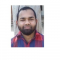## How to display outliers in boxplot with different shape in base R?Updated on 22-Nov-2021 08:19:41
To display outliers in boxplot with different shape in base R, we can use outpch argument in boxplot.For example, if we have a vector called X that contains some outliers then we can create a boxplot of X with different shape of outliers by using the below given command −boxplot(X,outpch=17)ExampleTo display outliers in boxplot with different shape in base R, use the code given below −x

## How to change the color of box of boxplot in base R?Updated on 22-Nov-2021 08:18:04
To change the color of box of boxplot in base R, we can use col argument inside boxplot function.For example, if we have a vector called V and we want to create a boxplot of V without red colored box then we can use the following command −boxplot(x,col="red")ExampleTo change the color of box of boxplot in base R, use the code given below −x

## How to change the color of outliers in base R boxplot?Updated on 22-Nov-2021 08:16:26
To change the color of outliers in base R boxplot, we can use outcol argument in boxplot function.For example, if we have a vector called X that contains some outliers then we can create a boxplot of X with blue color outliers by using the below given command −boxplot(X,outcol="red")ExampleTo change the color of outliers in base R boxplot, use the code given below: −x

## How to write plot description outside plot in facetted plot using ggplot2 in R?Updated on 22-Nov-2021 08:14:29
To write plot description outside plot using ggplot2, we can use annotate function and coord_cartesian function. The annotate function will define the plot description and the coord_cartesian function will define the position of the text outside the plot area.Check out the below given example to understand how it works.ExampleFollowing snippet creates a sample data frame −x

## How to test if strings stored in a vector are present in an R list?Updated on 22-Nov-2021 08:11:43
To test if strings stored in a vector are present in an R list or not, we can use mapply function. For example, if we have a vector of strings say V and a list called LIST then we can check whether elements in V are present in LIST by using the following command − mapply(%in%,V,LIST)Example 1Following snippet creates a vector and list −x

## Find the frequency of successive occurrences less than equal to a threshold in an R data frame column?Updated on 22-Nov-2021 08:09:28
To find the frequency of successive occurrences less than a threshold value in an R data frame column, we can use rle function along with sum function.Check out the below given examples to understand how it can be done.Example 1Following snippet creates a sample data frame −x

## How to find numbers that are divisible by a certain number for a range of values in R?Updated on 22-Nov-2021 07:53:15
In R, the divisibility of a number by a certain number can be found by using the modulus operator %%. If we want to check the divisibility of a set of numbers by a certain number then for loop will be used.Check out the below given examples to understand how it can be done.Example 1To check which numbers between 1 and 100 are divisible by 10, use the following code −for(x in 1:100){    + if(x%%10==0){          + print(x)    + } + }If you execute the above given code, it generates the following output −  10 ... Read More

## How to create a scatterplot with white background and no gridlines using ggplot2 in R?Updated on 22-Nov-2021 07:45:48
Practically, the scatterplots are well visualized on white background just like on white paper. If we want to create a scatterplot with white background and without gridlines using ggplot2 then we can apply classic theme to the plot.Check out the below given example to understand how it can be done.ExampleFollowing snippet creates a sample data frame −x

## How to find the number of unique values in comma separated strings stored in an R data frame column?Updated on 22-Nov-2021 07:41:06
If we have comma separated values that contains duplicate and unique values then we might want to find the number of unique values within each comma separated value. To find the unique values in comma separated strings stored in an R data frame column, we can use stri_extract_all_regex function of stringi package along with sapply function.Check out the below examples to understand how it can be done.Example 1Following snippet creates a sample data frame −x

## How to combine columns by excluding missing values in R?Updated on 22-Nov-2021 07:34:28
If we have a data set that contains missing values at alternate places for each column then we might want to combine the columns by excluding those missing values, this will reduce the data set and the analysis is likely to become easier.For this purpose, we can use na.exclude function along with apply function as shown in the below given examples.Example 1Following snippet creates a sample data frame −x1